# On statistical inference

library(FuzzySTs)

## boot.mean.ml(): Estimates the bootstrap distribution of the likelihood ratio LR

FuzzySTs::boot.mean.ml() estimates the empirical distribution of the likelihood ratio LR by the bootstrap technique as exposed in the PhD Thesis of Berkachy R. (“The signed distance measure in fuzzy statistical analysis”). It produces a vector of replications of LR for several random drawings from a primary data set using two algorithms written as Algorithms 1 and 2. The coefficient $$\eta$$ is then nothing but the $$1-\delta$$-quantile of this distribution. This function can till now be used to the following distributions: the normal, the Poisson and the Student distributions. The related density functions are known and their likelihood functions can be accordingly computed. In addition, this function computes internally the MLE-estimator by the EM-algorithm using the function EM.Fuzzy::EM.Trapezoidal() by the EM.Fuzzy package. A fuzzy number modelling the crisp estimator can be added. The default spread of this number is $$2$$.

The number of replications, the smoothness and the margins of calculations of the obtained distributions are defined by the nsim, step and the margin fixed by default to $$100$$, $$0.05$$ and $$c(5,5)$$ respectively.

# Calculation of the 95%-quantile eta of the bootstrapped distribution
mat <- matrix(c(1,2,2,2,2,1),ncol=1)
MF111 <- TrapezoidalFuzzyNumber(0,1,1,2)
MF112 <- TrapezoidalFuzzyNumber(1,2,2,3)
PA11 <- c(1,2)
data.fuzzified <- FUZZ(mat,mi=1,si=1,PA=PA11)
emp.dist <- boot.mean.ml(data.fuzzified, algorithm = "algo1", distribution = "normal",
sig = 0.05, nsim = 5, sigma = 1)
(eta.boot <- quantile(emp.dist,  probs = 95/100))
#>      95%
#> 2.268668

## fci.ml.boot(): Estimates a fuzzy confidence interval by the likelihood method and the bootstrap technique

FuzzySTs::fci.ml.boot() estimates the fuzzy confidence interval by the likelihood method given by the left and right $$\alpha$$-cuts, as exposed in the PhD Thesis of Berkachy R. (“The signed distance measure in fuzzy statistical analysis”). The proposed method can be used to compute the interval without a specific expression for a particular distribution to estimate a given related parameter. However, for our current situation, we restrict ourselves to distributions drawn from the normal, the Poisson and the Student distribution since the related density functions are known and their likelihood functions can be easily computed. An eventual upgrade to this function is welcomed in order to be able to introduce empirical density functions as instance. In addition, we have used the bootstrap technique to estimate the distribution of the likelihood ratio. This task is done using the function FuzzySTs::boot.mean.ml() in the purpose of estimating the quantile $$\eta$$. The smoothness and the margins of calculations of the constructed interval are defined by the step and the margin fixed by default to $$0.05$$ and $$c(5,5)$$.

# Calculation of the 95% fuzzy confidence interval by the likelihood method
# and using the bootstrap technique
data <- matrix(c(1,2,3,2,2,1,1,3,1,2),ncol=1)
MF111 <- TrapezoidalFuzzyNumber(0,1,1,2)
MF112 <- TrapezoidalFuzzyNumber(1,2,2,3)
MF113 <- TrapezoidalFuzzyNumber(2,3,3,4)
PA11 <- c(1,2,3)
data.fuzzified <- FUZZ(data,mi=1,si=1,PA=PA11)
Fmean <- Fuzzy.sample.mean(data.fuzzified)
emp.dist <- boot.mean.ml(data.fuzzified, algorithm = "algo1", distribution = "normal",
sig = 0.05, nsim = 5, sigma = 0.79)
coef.boot <- quantile(emp.dist,  probs = 95/100)
head(fci.ml.boot(data.fuzzified, t = Fmean, distribution = "normal", sig= 0.05, sigma = 0.62,
coef.boot = coef.boot))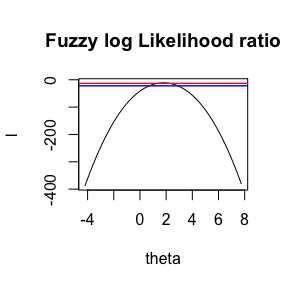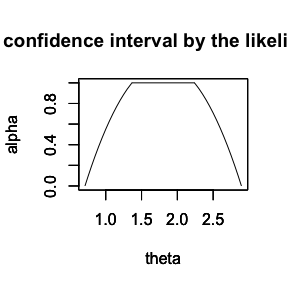#>           [,1]     [,2]
#> [1,] 0.7102729 2.894549
#> [2,] 0.7148913 2.889917
#> [3,] 0.7195097 2.885286
#> [4,] 0.7241282 2.880654
#> [5,] 0.7287466 2.876022
#> [6,] 0.7333650 2.871391

## Fuzzy.decisions(): Computes the fuzzy decisions of a fuzzy inference test by the traditional fuzzy confidence intervals

FuzzySTs::Fuzzy.decisions() calculates the fuzzy decisions obtained from a fuzzy inference test based on the fuzzy confidence intervals as described in the PhD Thesis of Berkachy R. (“The signed distance measure in fuzzy statistical analysis”). The corresponding construction of these decisions are also shown. We have to mention that for this function, all the cases of intersection between the fuzzy confidence intervals and the fuzzy null hypothesis in the two-sided and one-sided cases are taken into account. In addition, by this function, one could get the defuzzification of the obtained fuzzy decisions. This task can be made by calculating the distance of these fuzzy numbers to the fuzzy origin, given by the function FuzzySTs::distance() for which any distance of the family of distances can be used. Note that for the likelihood method, an analog function is called $\text{Fuzzy.decisions.ML (data.fuzzified, H0, H1, t, coef.boot, mu=NA, sigma=NA, etc)},$ where we introduce a parameter coef.boot which is a decimal representing the (1-sig)-quantile of the bootstrap distribution of the likelihood ratio, calculated using the function FuzzySTs::boot.mean.ml() and stats::quantile().

# Calculation of fuzzy decisions using the function Fuzzy.decisions
H0 <- alphacut(TriangularFuzzyNumber(2.9,3,3.1), seq(0,1, 0.01))
H1 <- alphacut(TriangularFuzzyNumber(3,3,5), seq(0,1,0.01))
t <- alphacut(TriangularFuzzyNumber(0.8,1.80,2.80), seq(0,1,0.01))

res <- Fuzzy.decisions(type = 0, H0, H1, t = t, s.d = 0.79, n = 10, sig = 0.05,
distribution = "normal", distance.type = "GSGD")

res$RH0 #> Trapezoidal fuzzy number with: #> support=[0.67818,0.736693], #> core=[0.710362,0.710362]. res$DRH0
#> Trapezoidal fuzzy number with:
#>    support=[0.210709,0.354216],
#>       core=[0.289638,0.289638].
res$D.RH0 #>  0.70905 res$D.DRH0
#>  0.2882915

# Calculation of fuzzy decisions using the function Fuzzy.decisions.ML
data <- matrix(c(1,2,3,2,2,1,1,3,1,2),ncol=1)
MF111 <- TrapezoidalFuzzyNumber(0,1,1,2)
MF112 <- TrapezoidalFuzzyNumber(1,2,2,3)
MF113 <- TrapezoidalFuzzyNumber(2,3,3,4)
PA11 <- c(1,2,3)
data.fuzzified <- FUZZ(data,mi=1,si=1,PA=PA11)

H0 <- alphacut(TriangularFuzzyNumber(2.9,3,3.1), seq(0,1, 0.01))
H1 <- alphacut(TriangularFuzzyNumber(3,3,5), seq(0,1,0.01))
t <- alphacut(TriangularFuzzyNumber(0.8,1.80,2.80), seq(0,1,0.01))

emp.dist <- boot.mean.ml(data.fuzzified, algorithm = "algo1", distribution = "normal",
sig = 0.05, nsim = 5, sigma = 0.79)
coef.boot <- quantile(emp.dist,  probs = 95/100)
res <- Fuzzy.decisions.ML(data.fuzzified, H0, H1, t = t, coef.boot = coef.boot, sigma = 0.79,
sig = 0.05, distribution = "normal", distance.type = "GSGD")

res$RH0 #>  1 res$DRH0
#>  0
res$D.RH0 #>  1 res$D.DRH0
#>  0

## Fuzzy.CI.test(): Computes a fuzzy inference test by the traditional fuzzy confidence intervals

FuzzySTs::Fuzzy.CI.test() tests a fuzzy null hypothesis against a fuzzy alternative one, based on traditional fuzzy confidence intervals. This test is computed using the function FuzzySTs::Fuzzy.decisions() which provides the fuzzy decisions related to the test. These decisions are afterwards defuzzified by calculating their distance to the fuzzy origin, with respect to the family of distances given by the function FuzzySTs::distance(). Note that this function is made for the case of the mean only. This function is designed to be used with the normal, the Poisson and the Student distributions.

# Calculation of a a fuzzy hypotheses test by the traditional fuzzy confidence interval
H0 <- TriangularFuzzyNumber(2.9,3,3.1)
H1 <- TriangularFuzzyNumber(3,3,5)

res <- Fuzzy.CI.test(type = 0, H0, H1, t = TriangularFuzzyNumber(0.8,1.80,2.80), s.d = 0.79,
n = 10, sig = 0.05, distribution = "normal", distance.type="GSGD")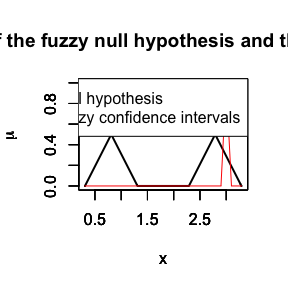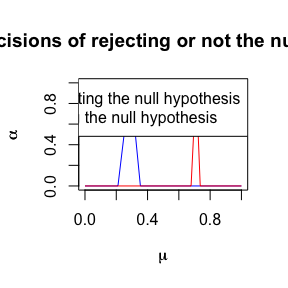res$decision #>  The signed distance 0.2883 of not rejecting the null hypothesis H0 is smaller than the signed distance 0.7091 of rejecting it. Decision: The null hypothesis (H0) is rejected at the 0.05 significance level. res$RH0
#> Trapezoidal fuzzy number with:
#>    support=[0.67818,0.736693],
#>       core=[0.710362,0.710362].
res$DRH0 #> Trapezoidal fuzzy number with: #> support=[0.210709,0.354216], #> core=[0.289638,0.289638]. res$D.RH0
#>  0.70905
res$D.DRH0 #>  0.2882915 ## Fuzzy.CI.ML.test(): Computes a fuzzy inference test by the fuzzy confidence intervals method calculated by the Likelihood method and the bootstrap technique FuzzySTs::Fuzzy.CI.ML.test() tests a fuzzy null hypothesis against a fuzzy alternative one, based on the fuzzy confidence interval constructed using the likelihood method. The bootstrap technique is used for the estimation of the likelihood ratio distribution. The constructed confidence interval is computed using the function FuzzySTs::fci.ml.boot() for the computation of the confidence interval and FuzzySTs::Fuzzy.decisions.ML() for the computation of the fuzzy decisions. These latter are then defuzzified by a distance chosen from the family of distances given by the function FuzzySTs::distance(). The use of the function FuzzySTs::Fuzzy.CI.ML.test() is restricted to the distributions drawn from the normal, the Poisson and the Student distribution. An eventual improvement of these functions is to consider the empirical distributions, or any other known distribution. # Calculation of a fuzzy hypotheses test by the fuzzy confidence interval # using the likelihood method and the bootstrap technique data <- matrix(c(1,2,3,2,2,1,1,3,1,2),ncol=1) MF111 <- TrapezoidalFuzzyNumber(0,1,1,2) MF112 <- TrapezoidalFuzzyNumber(1,2,2,3) MF113 <- TrapezoidalFuzzyNumber(2,3,3,4) PA11 <- c(1,2,3) data.fuzzified <- FUZZ(data,mi=1,si=1,PA=PA11) Fmean <- Fuzzy.sample.mean(data.fuzzified) H0 <- TriangularFuzzyNumber(2.2,2.5,3) H1 <- TriangularFuzzyNumber(2.5,2.5,5) emp.dist <- boot.mean.ml(data.fuzzified, algorithm = "algo1", distribution = "normal", sig= 0.05, nsim = 5, sigma = 0.7888) coef.boot <- quantile(emp.dist, probs = 95/100) res <- Fuzzy.CI.ML.test(data.fuzzified, H0, H1, t = Fmean, sigma=0.7888, coef.boot = coef.boot, sig=0.05, distribution="normal", distance.type="GSGD")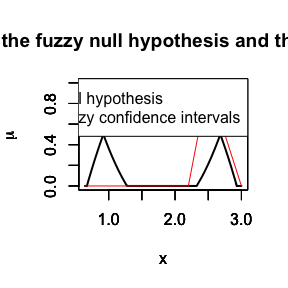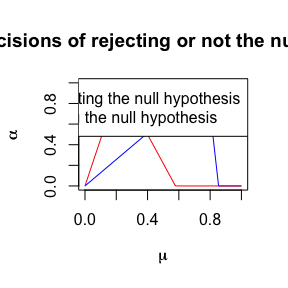res$RH0
#> Trapezoidal fuzzy number with:
#>    support=[0,0.578325],
#>       core=[0.220381,0.220381].
res$DRH0 #> Trapezoidal fuzzy number with: #> support=[0,0.853986], #> core=[0.779619,0.779619]. res$decision
#>  The signed distance 0.64 of not rejecting the null hypothesis H0 is bigger than the signed distance 0.2929 of rejecting it.  Decision: The null hypothesis (H0) is not rejected at the 0.05 significance level.

## Fuzzy.p.value(): Computes the fuzzy p-value of a given fuzzy hypothesis test

FuzzySTs::Fuzzy.p.value() calculates the fuzzy p-value of a given hypothesis test as presented in the PhD Thesis of Berkachy R. (“The signed distance measure in fuzzy statistical analysis”). For this fuzzy p-value, the null and the alternative hypotheses have to be defined, as well as the corresponding parameter (the mean in the proposed example), and the considered distribution. The normal, the Poisson and the Student distributions can be used. We add that a defuzzification of the obtained fuzzy number is proposed also. This task can be done by choosing a distance from the family of distances given in FuzzySTs::distance().

# Calculation of a fuzzy p-value of a fuzzy hypotheses test

H0 <- TriangularFuzzyNumber(2.2,2.5,3)
H1 <- TriangularFuzzyNumber(2.5,2.5,5)

Fuzzy.p.value(type=1, H0, H1, t=TriangularFuzzyNumber(0.8,1.8,2.8),
s.d=0.7888, n=10, sig=0.05, distribution="normal", distance.type="GSGD")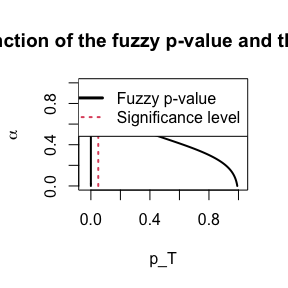#>  Defuzzified p-value =  0.326619
#>  The null hypothesis (H0) is not rejected with the degree of conviction D = 0.326619  at the  0.05  significance level.